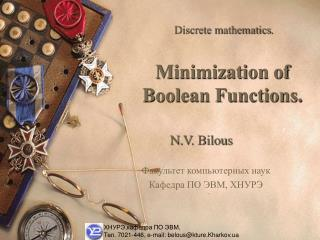DownloadDownload PresentationMinimization of Boolean Functions.

# Minimization of Boolean Functions.

Télécharger la présentation## Minimization of Boolean Functions.

- - - - - - - - - - - - - - - - - - - - - - - - - - - E N D - - - - - - - - - - - - - - - - - - - - - - - - - - -
##### Presentation Transcript

1. Discrete mathematics. Minimization of Boolean Functions. N.V. Bilous Факультеткомпьютерных наук Кафедра ПО ЭВМ, ХНУРЭ ХНУРЭ,кафедра ПО ЭВМ, Тел. 7021-446, e-mail: belous@kture.Kharkov.ua

2. Criterion of minimization. The task of minimization is to find the simplest formula according to chosen criterion of minimization among the set of formulae corresponding to given Boolean function.

3. The Karnaugh map The Karnaugh map is a graphical method for finding terms to combine for Boolean functions involving a relatively small number of variables.

4. The Karnaugh map Cells are said to be adjacent if the minterms that they represent differ in exactly one literal. For instance, the cell representing is adjacent to the cells representing and .

5. The rule of gluing contours of the Karnaugh Maps. • Build Karnaugh map corresponding to given function. • The cells are united in groups defining the operations of gluing. Only adjacent cells in which the unities are situated take part in the unification. • Only the number of cells equal to 2n, n=1,2,3,... may be united in group. In this case group may be have only rectangle or square form.

6. The rule of gluing contours of the Karnaugh Maps. • The task of gluing consists in finding the collection of maximum groups. Maximum groupis a group which does not completely belong to any other group and corresponds toelementary implicant of function. • Each group obtaining after gluing corresponds to implicant of function. • Disjunction of all obtaining elementary implicantis the result ofminimization formula and it is minimal DNF.

7. The Karnaugh Map for Three Variables. The Karnaugh maps for three variables have the form of table 24 where the columns corresponds to different collections of values the first two variables, and the lines – to values 0 an 1 the third variable.

8. The Karnaugh Map for Three Variables. Example. Build the Karnaugh map for function

9. The Karnaugh Map for Three Variables. The operation of gluing may be supplied to minterms corresponding to any two adjacent cells as they are different by just one variable. Cells connecting with cells A and B

10. The Karnaugh Map for Three Variables. Example. Find MDNF for function Solution. Build the Karnaugh map for given function

11. The Karnaugh Map for Four Variables. The Karnaugh maps for four variables has the size 44. Each cell has four adjacent cells. The rules of gluing cells and noting the resulting formula are previous. The difference is that not only the border right and border left columns are necessary to count but border upper and border lower lines too.

12. The Karnaugh Map for Four Variables. Example. Build MDNF for function

13. The Karnaugh Map for Four Variables. Solution. Build the Karnaugh map.

14. The Karnaugh Map for Five Variables. The Karnaugh maps for five variables represents as two-layer parallelepiped in space where each layer corresponds to Karnaugh map from the first four variables. Each cell in the Karnaugh maps for five variables has five adjacent cells: four on its layer of map and the fifth on the adjacent, i.e. the cell which coincides with given if the layers of map place one above the other.

15. The Karnaugh Map for Five Variables. Example. Build MDNF for function.

16. The Karnaugh Map for Five Variables. Solution. Example

17. The Karnaugh Map for Six Variables. The Karnaugh maps for six variables represents as four-layer parallelepiped in space where each layer corresponds to Karnaugh map from the first four variables. The layers of map are considered adjacent if interpretation of the fifth and the sixth variables corresponding to them differs only by one literal.

18. The Karnaugh Map for Six Variables. Each cell in the Karnaugh maps for six variables has six adjacent cells: four on its layer of map and two on the adjacent layers of map. The cells coincidewith given if the layers of map place one above the other.

19. The Karnaugh Map for Six Variables. Example. Build MDNF for function.

20. The Karnaugh Map for Six Variables. Solution. Given function equals to unit in the following interpretations: (0,0,1,0,0,0), (0,0,1,0,0,1), (0,0,1,0,1,0), (0,0,1,0,1,1), (0,1,0,1,0,0), (0,1,0,1,0,1), (0,1,1,1,0,0), (0,1,1,1,0,1), (1,1,0,1,0,0), (1,1,1,1,0,1), (1,1,0,1,1,0), (1,1,0,1,0,1), (1,1,1,1,0,0). Build the Karnaugh map corresponding to given function.

21. The Karnaugh Map for Six Variables. Continuation of example.

22. Don't care conditions. If for the decisionof task not all combinations of input data are used then any value of function in non-using interpretations may be arbitrarily chosen and values of the function for these combinations are called don't care conditions.

23. Don't care conditions. Example. Functionf(x,y,z,t) equals to unit ininterpretations (0,0,1,0), (0,1,1,0), (1,0,1,0), (1,0,0,0) and is indefinite if xy=1. We need to build MDNF. Solution.